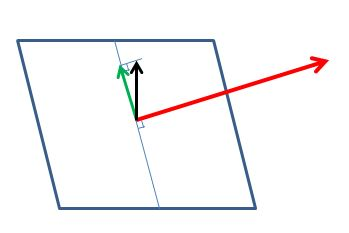# Path3D

## How To Use Path3D

A Path3D is a mathematical object created from a sequence of position vectors of points on a curve. Once obtained a Path3D can be used to determine the triplet of vectors tangent, normal and binormal of the curve for each of those points. Each triplet can then be used as a local system coordinate. You could set, for example, the camera direction to the normal as it follows the curve.

A Path3D object is simple to construct as follows

javascript
const points = [v1, v2, ..., vn];          // array of Vector3const path3d = new BABYLON.Path3D(points);

You can then get the array of tangents, normals and binormals as follows

javascript
const tangents = path3D.getTangents();const normals = path3D.getNormals();const binormals = path3D.getBinormals();

each element of the arrays is a Vector3 .

Tangents, Normals, and Binormals

Please zoom in and rotate : tangents in red, normals in blue, binormal in green.

Notice, in the next example, how the the triplets slightly rotate when the curve goes more into depth.

Tangents, Normals, and Binormals - Color Coded

Whilst at any point on the curve there is only one tangent there can be an infinite number of normals and hence binormals. If the default one does not suit you it is possible to set the normal direction

## Path3D Methods

As well as getTangents, getNormals and getBinormals there are some other methods of Path3D.

### Get Curve Points

The getCurve method returns a copy of the initial Vector3 array given to create the Path3D object.

javascript
const curvePoints = path3d.getCurve();

### Get Distances

The getDistances method returns an array of distances from one curve point to the next in order of points and with 0 being the first distance.

For the array of points [(1, 0, 0), (5, 0, 0), (5, 1, 0)] the array of distances is [0, 4, 5].

javascript
const distances = path3d.getDistances();

### Interpolation

You can get info about any virtual point (from 0.0 to 1.0) along the path by functions that interpolate between the path points. Those are the following:

getPointAt, getTangentAt, getNormalAt, getBinormalAt, getDistanceAt, getPreviousPointIndexAt, getSubPositionAt

### Copying (part of) the path

The slice method returns a new Path3D that is subpath (slice) of the original path. It takes a start and end position from 0.0 to 1.0, or negative values, which wrap back around from 1.0

### Update

In order to avoid memory re-allocation (when in the render loop for example) since the given points array is internally copied, you can update an existing Path3D object with its update() method.

javascript
const points1 = [v1, v2, ..., vn];          // array of Vector3const path3d = new BABYLON.Path3D(points1);const points2 = [u1, u2, ..., un];          // another array of Vector3path3D.update(points2);

Tangents, normals and bi-normals are thus recomputed for this new path.

Update Path3D

### Getting the closest position to a point

The getClosestPositionTo function returns the position, from 0.0 to 1.0, of the closest virtual point along the path to an arbitrary Vector3.

## Set The Normal

For any vector there are an infinite number of vectors at right angles to it.In creating a Path3D object Babylon.js assigns a default direction for the normal. If you want to set the direction of the normal yourself, you need to pass a vector for a second parameter to Path3D on creation or on update.

javascript
const initialVector = new BABYLON.Vector3(0, 1, 0);const otherVector = new BABYLON.Vector3(0, 0, 1);const points = [v1, v2, ..., vn];          // array of Vector3const path3d = new BABYLON.Path3D(points, initialVector);// do stuff ...path3d.update(points, otherVector);

The normal will be the projection of your parameter vector onto the plane orthogonal to the tangent at the point position.

As can been in the diagram below, when the parameter is a vertical vector (black), this is projected onto the plane orthoganal to the tangent vector (red) to form the normal (green).The playground example shows what happens as the vector setting the normal direction is rotated.

Path3D with Rotating Normals

## Normalization and tangent alignment

Apart from the first normal, there are two more parameters:

raw, boolean, false by default. If true the tangents, normals and binormals aren't normalized. Useful to depict path acceleration or speed.

alignTangentsWithPath, boolean, false by default. If true the tangents will be aligned with the path.

## Objects Following A Path

As mentioned in the beginning section, the position of points along the curve, together with the tangent, normal, and binormal vectors, can be used to guide the position and orientation of an object, creating interesting yet effortless animations.

Camera Following a Path

The playground above illustrates this technique. The Path3D is constructed as the combination of a few cubic Bèzier curves (but manually defining the points would work just fine). On each point of the path, its tangent and binormal vectors are passed as arguments to the FromLookDirectionRH() method of the Quaternion class, orienting the camera such that it looks down the tangent direction, and points up towards the binormal. We then set the sequences of positions and orientations as animation keys, and play it. This idea isn't limited to Cameras, as it could also be used to define paths along which characters would walk in a game, for example.